# How to create a Trigger Condition

The second part of the Dynamic model is creating Trigger Conditions:

1. Select the "+ Add Condition" button.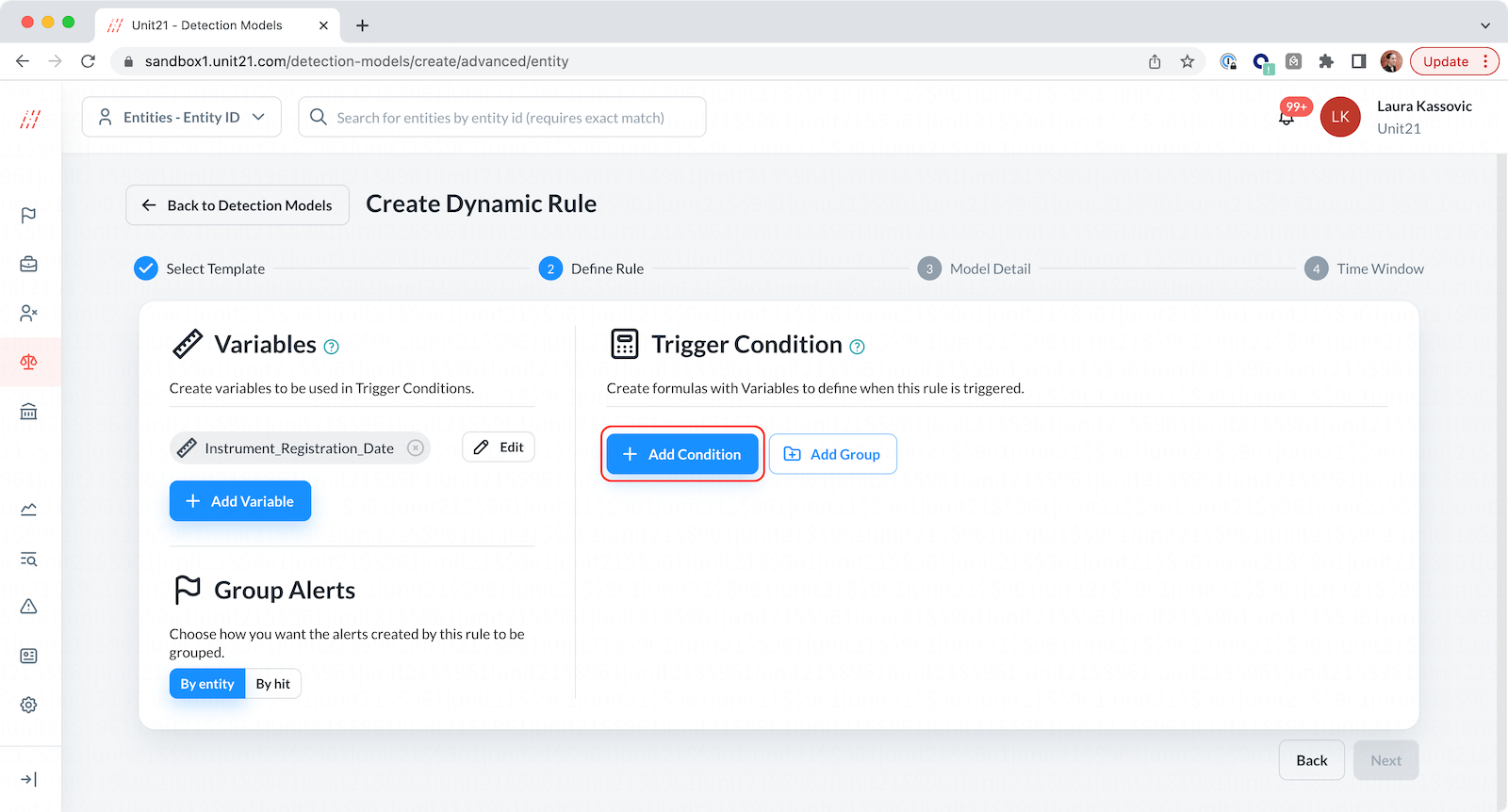1. This will prompt the Text Formula Builder where mathematical operators can be used on the Variables you created previously. You can select the basic formula or the advanced formula: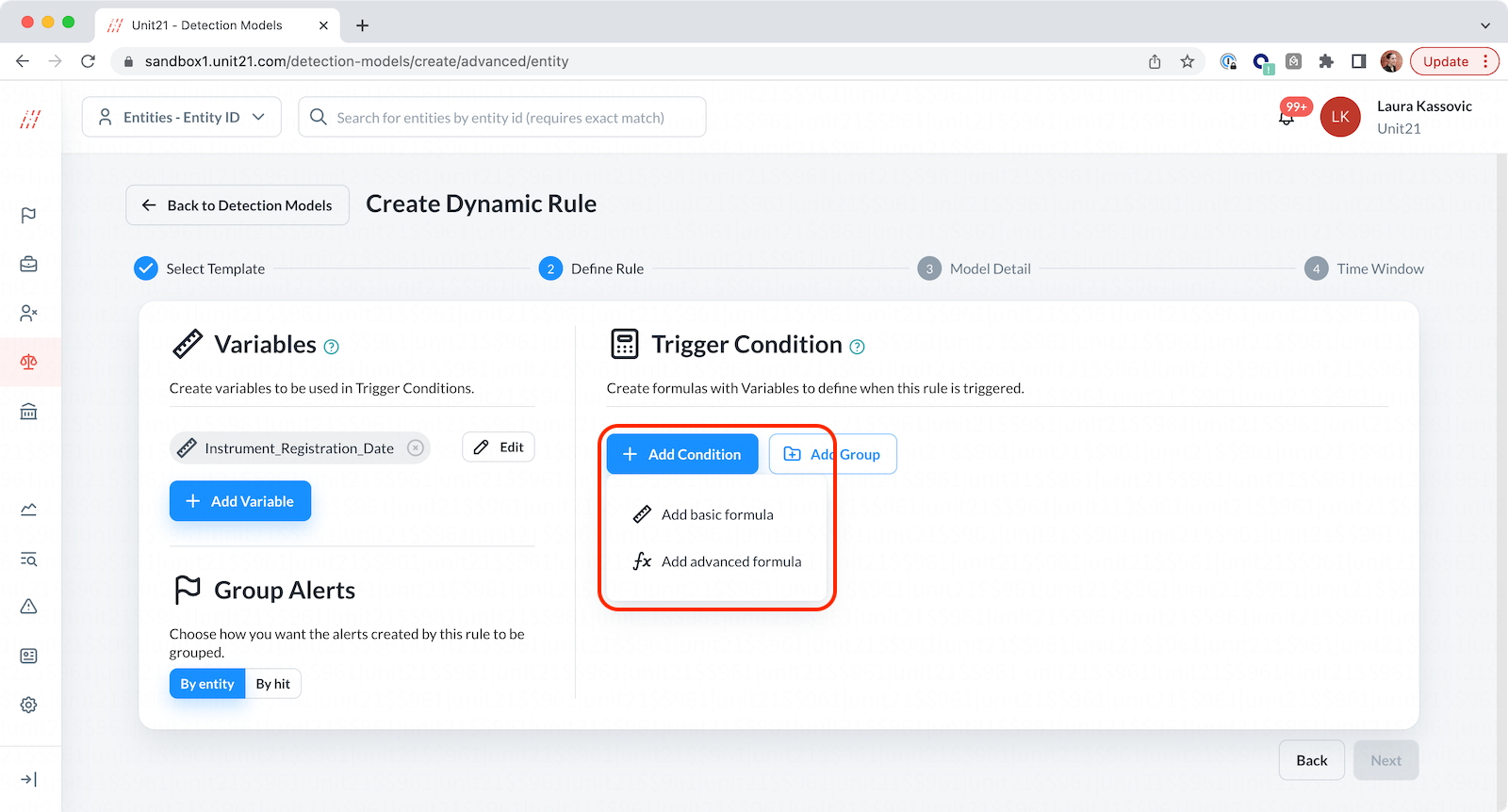The basic formula allows agents to create variable logic using dropdowns: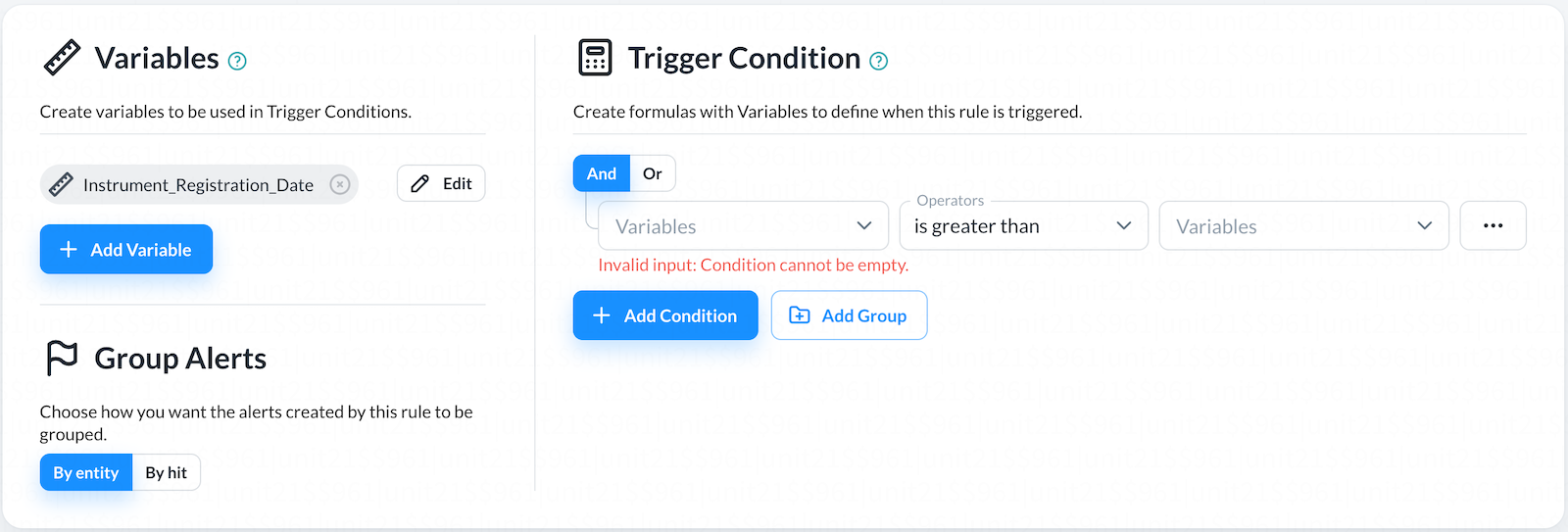The advanced formula is free-form text: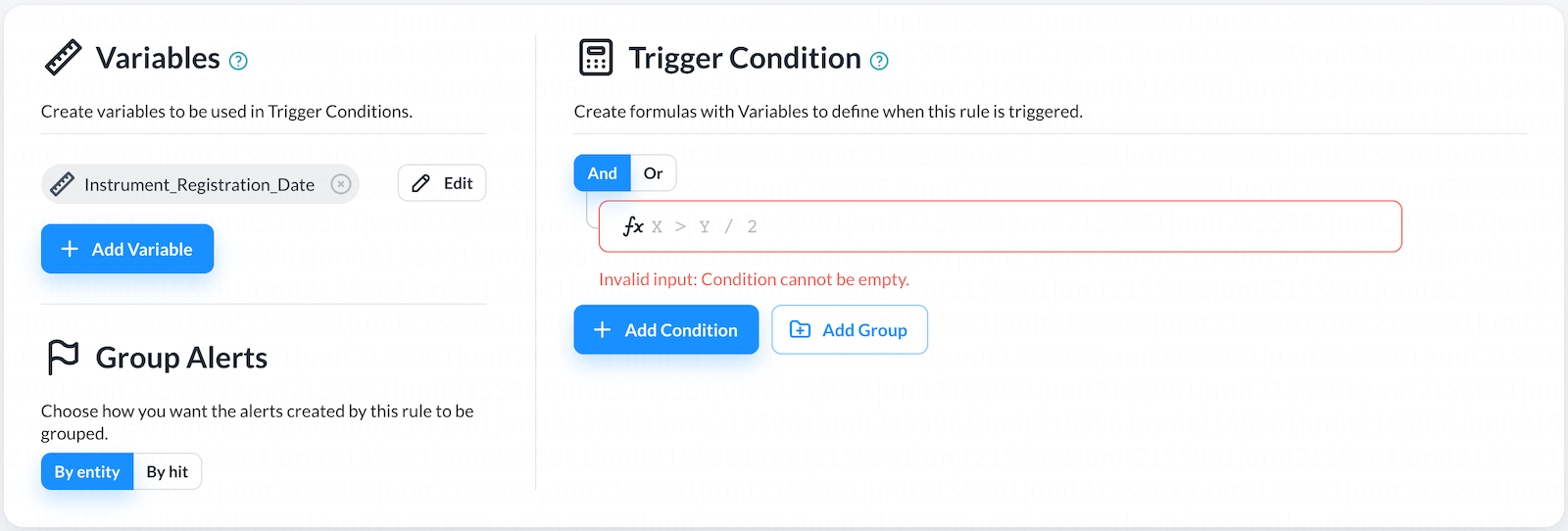1. Let's take a look at the basic formula builder in detail: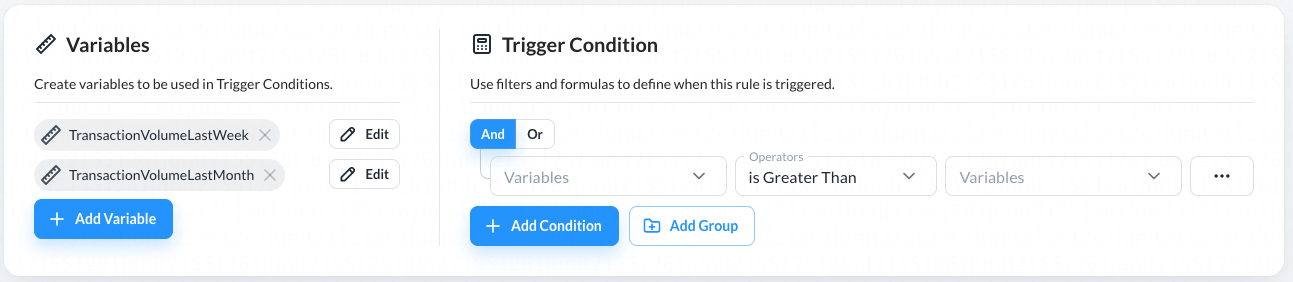All the Variables you previously created are available in the Variables dropdown: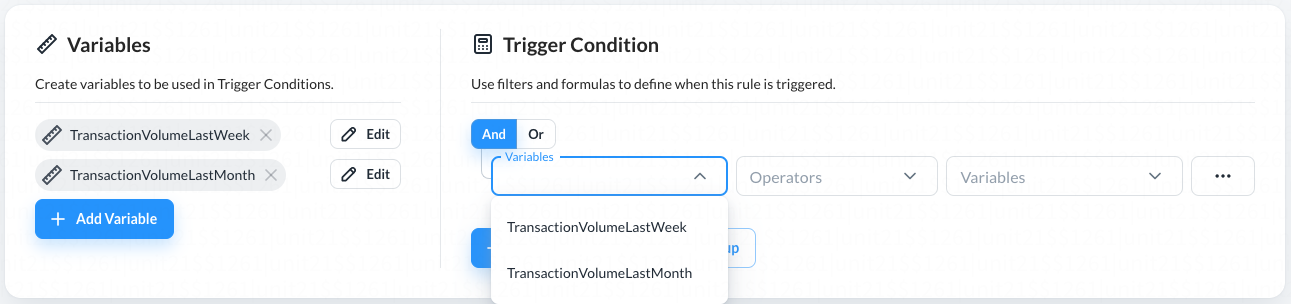The mathematical operands are available in the Operators dropdown: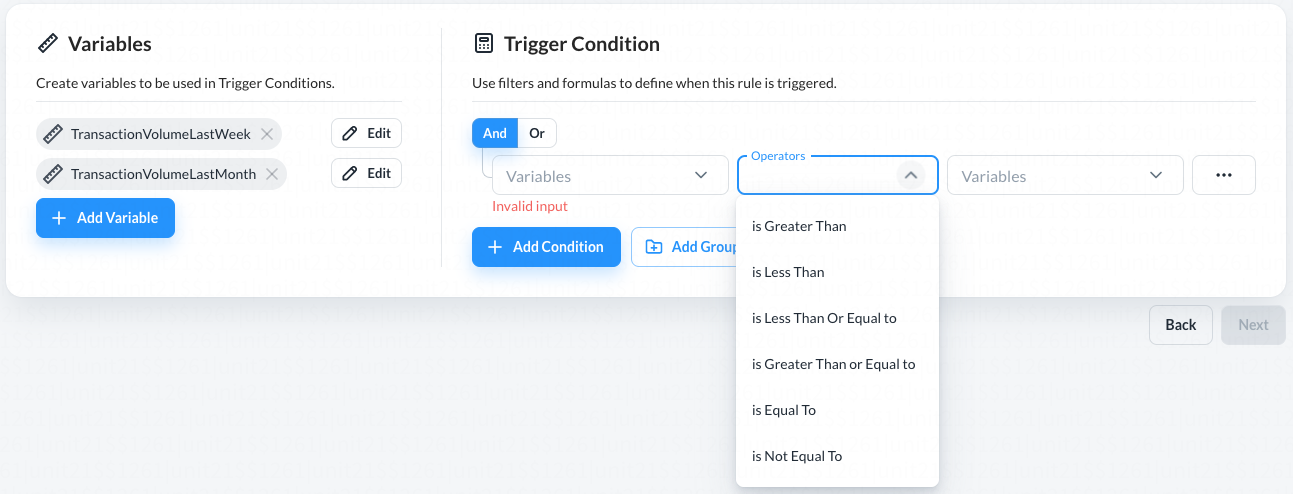Therefore a formula for basic comparative logic between Variables can be achieved: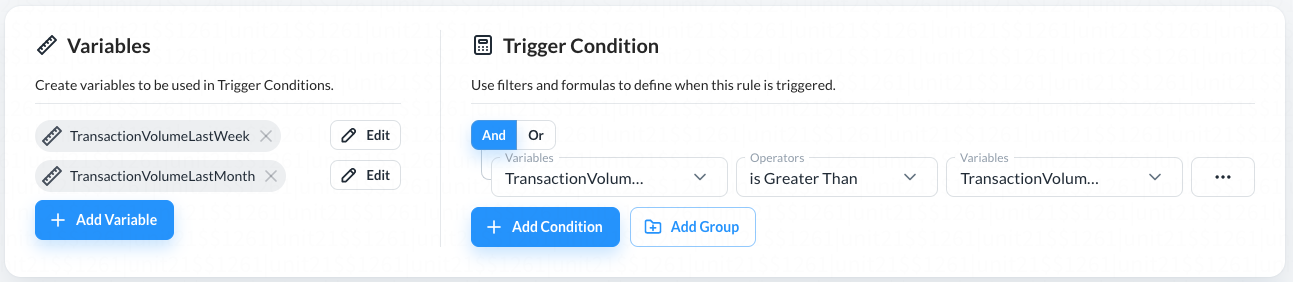1. The logic can be converted to text using the Convert to Text button in the ... menu: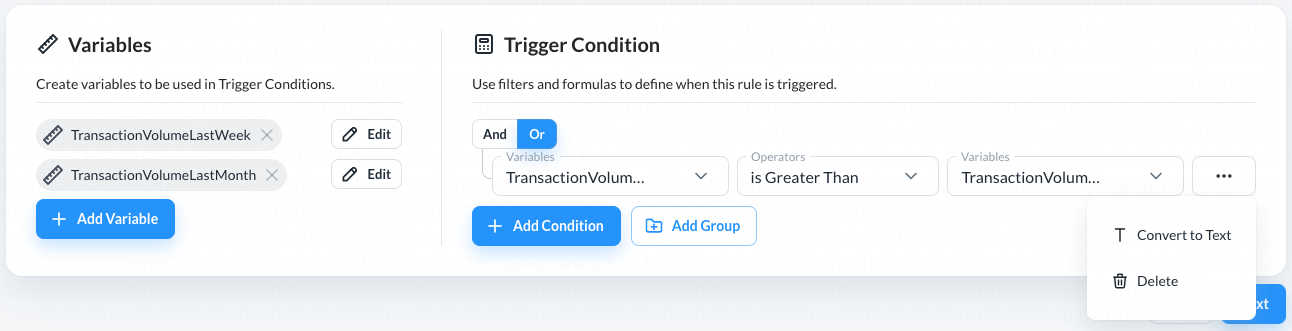You can also use this menu to Delete any logic.

1. Convert to Text also reveals the Advanced formula builder: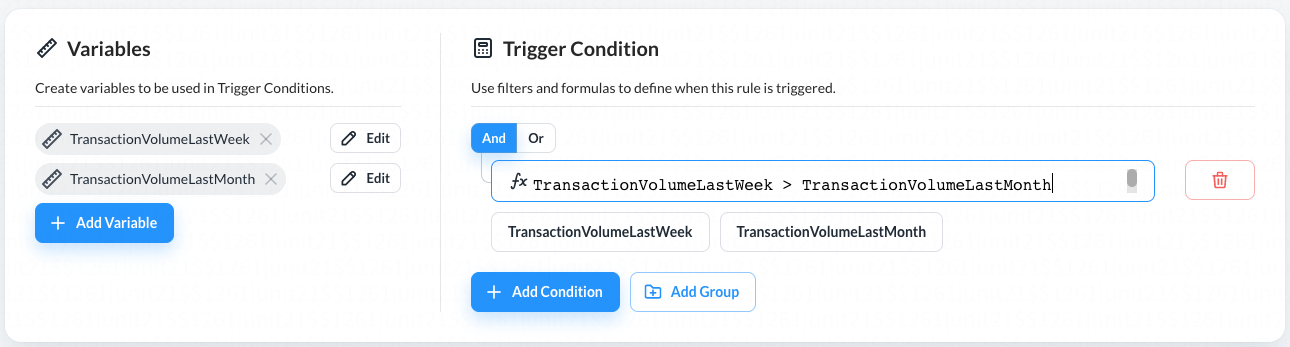In text mode, more complicated logic can be created. For example, the Variables can be divided or multiplied: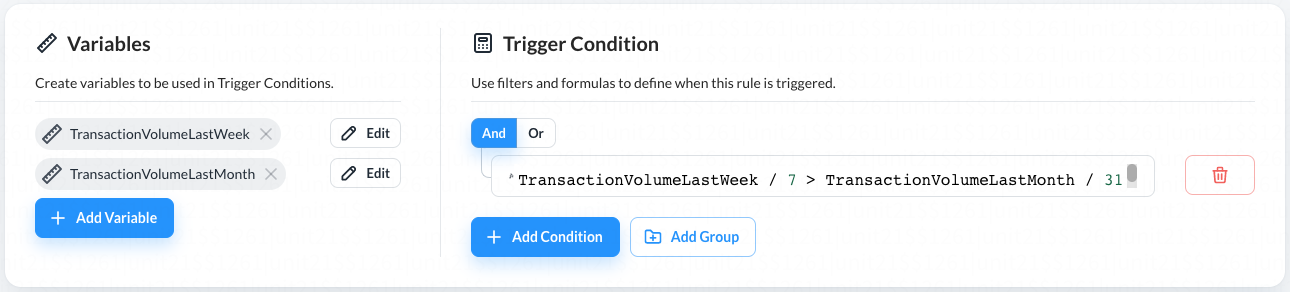1. Multiple Triggers can be AND'ed or OR'ed together to create a more complicated Formula: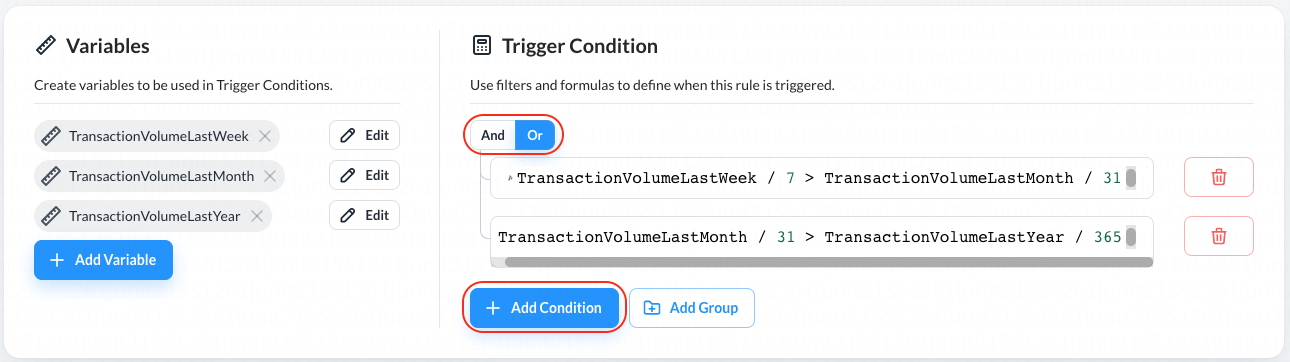So in the example above, having two trigger conditions:

• A: TransactionVolumeLastWeek / 7 > TransactionVolumeLastMonth / 31
• B: TransactionVolumeLastMonth / 31 > TransactionVolumeLastYear / 365

Selecting And is similar to saying:

• A and B must be met to alert

Selecting Or is similar to saying:

• A or B must be met to alert